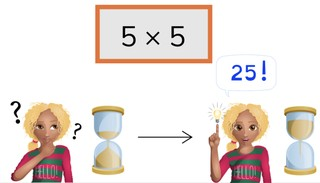Automaticity 5 times table

# Automaticity 5 times table

Students learn to memorize the 5 times table.8,000 schools use Gynzy92,000 teachers use Gynzy1,600,000 students use Gynzy

## General

The students learn to memorize the 5 times table.

## Standards

CCSS.Math.Content.3.OA.C.7

## Learning objective

Students will be able to memorize the 5 times table and quickly give the products.

## Introduction

Discuss with the students how the table should be filled in and drag the answers to the correct places.

## Instruction

First, have the students calculate the product from the 5 times table within 5, 4, and 3 seconds. Show the pile of cards. Explain that you are going to start the timer and drag the cover away to show the first problem. Have the students quickly give the correct answer to the problem, then drag the card to the side to show the next problem. Try to complete this within the time given by the timer.

Check whether the students have memorized the 5 times table with the following question:
- Why is it important to memorize the 5 times table?

## Quiz

The students practice the 5 times table in 3 timed exercises.

## Closing

Discuss once again the importance of memorizing the 5 times table. To close, you discuss with the students which product of the 5 times table belongs with each answer.

## Teaching tips

Give students who have difficulty memorizing the 5 times table a printed 5 times table to practice with. Have them practice in pairs with giving products from the 5 times table in shorter and shorter amounts of time.

## Instruction materials

You may use a printed 5 times table.

### The online teaching platform for interactive whiteboards and displays in schools

• Save time building lessons

• Manage the classroom more efficiently

• Increase student engagement